ISEE Primary 3 Math : ISEE Primary 3 Math

Example Questions

← Previous 1

Example Question #1 : Number Sense And Operations

Fill in the blank with the correct symbol.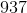__________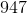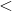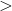Explanation:

Thesign means less than.is less thanbecause of the number in the tens place.is less than, which makesless thanExample Question #2 : Number Sense And Operations

If I havequarters andpennies, how many cents do I have?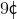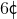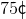Explanation:

Each quarter is worth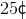and each penny is worth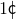.

We have three quarters and three pennies.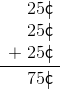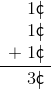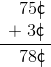Example Question #1 : Geometric Concepts

Fill in the blank.

This square provided is split into __________.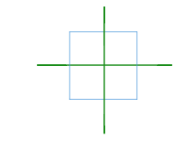fifths

thirds

halves

fourths

fourths

Explanation:

The square is split into four pieces, which means it is split into fourths.

Example Question #1 : Isee Primary 3 Math

Which would make the most sense to use if we were going to measure a bug?

Meter stick

Yardstick

Ruler

Measuring tape

Ruler

Explanation:

You would use a ruler to measure a bug because a bug is most likely smaller thaninches, which is the size of a ruler. All of the other tools would be used to measure much larger objects.

Example Question #2 : Isee Primary 3 Math

What time does the clock show?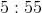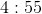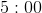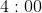Explanation:

When the hour hand (the shorter hand) points to a number on the clock, or is just past the number before it gets to the next number, we say that number as the hour. When the minute hand (the longer hand) points to a number on the clock we don't say that exact number.

Look at the clock below. For the minute hand, we count bys.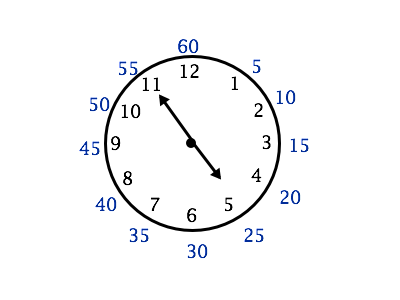This clock is showingfor the hour (it is past the numberbut not at theyet) and the minute hand is pointing at the, which meansminutes.

Our time isExample Question #3 : Isee Primary 3 Math

Mrs. Ryan's class had their class election today for their class president. The results are displayed in the chart below.

Use the graph below to help answer the question.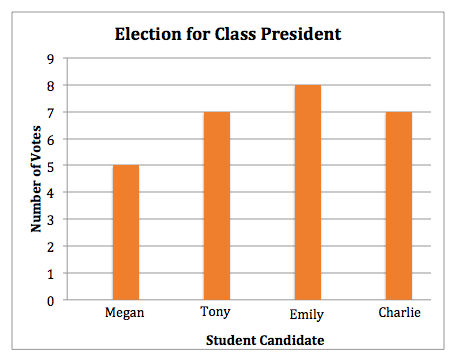How many people voted for Megan?Explanation:

The bar for Megan is the first bar on the left. The bar raises to the number, which meanspeople voted for Megan.

Example Question #4 : Isee Primary 3 Math

Kate's class is going to plant flowers outside of their class window. To help decide what flower to plant, her teacher took a class vote.

Use the graph below to help answer the question.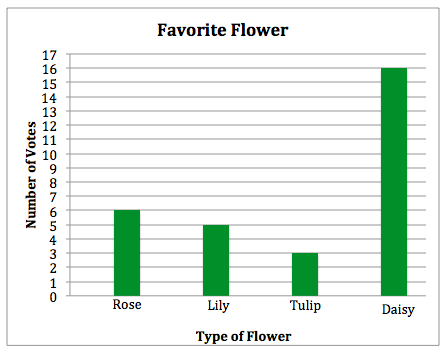What is the label of the x-axis?

Favorite Flower

Type of Flower

Kate's Class

Type of Flower

Explanation:

A graph is made up of an x-axis and a y-axis. The x-axis of a graph is always the horizontal line (a line that runs from left to right) and the y-axis is alway the vertical line (a line that runs from top to bottom)

The x-axis of this graph is labeled "Type of Flower".

Example Question #5 : Isee Primary 3 Math

The swing set isinches tall and the tree is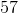inches tall. How much taller is the tree than the swing set?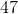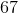Explanation:

This is a subtraction problem because we want to know how much taller the tree is compared to the swing set, or the difference in their height.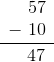Example Question #6 : Isee Primary 3 Math

When counting bys, what number comes after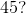Explanation:

When counting bys, we addto our previous number.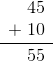Example Question #7 : Isee Primary 3 Math

What is the equation that shows the sum of the squares represented by this rectangular array?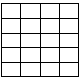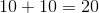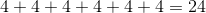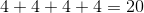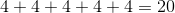When we count the number of squares in this picture we get. We haverows withsquares in each row. Our math equation for this picture could be written as